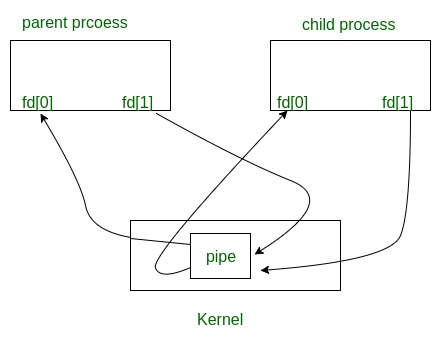GFG App
Open AppBrowser
Continue

# pipe() System call

Prerequisite : I/O System calls

Conceptually, a pipe is a connection between two processes, such that the standard output from one process becomes the standard input of the other process. In UNIX Operating System, Pipes are useful for communication between related processes(inter-process communication).

• Pipe is one-way communication only i.e we can use a pipe such that One process write to the pipe, and the other process reads from the pipe. It opens a pipe, which is an area of main memory that is treated as a “virtual file”.
• The pipe can be used by the creating process, as well as all its child processes, for reading and writing. One process can write to this “virtual file” or pipe and another related process can read from it.
• If a process tries to read before something is written to the pipe, the process is suspended until something is written.
• The pipe system call finds the first two available positions in the process’s open file table and allocates them for the read and write ends of the pipe.
• ```int pipe(int fds);

Parameters :
fd will be the fd(file descriptor) for the
fd will be the fd for the write end of pipe.
Returns : 0 on Success.
-1 on error.```

Pipes behave FIFO(First in First out), Pipe behave like a queue data structure. Size of read and write don’t have to match here. We can write 512 bytes at a time but we can read only 1 byte at a time in a pipe.

 `// C program to illustrate ` `// pipe system call in C ` `#include ` `#include ` `#define MSGSIZE 16 ` `char``* msg1 = ``"hello, world #1"``; ` `char``* msg2 = ``"hello, world #2"``; ` `char``* msg3 = ``"hello, world #3"``; ` ` `  `int` `main() ` `{ ` `    ``char` `inbuf[MSGSIZE]; ` `    ``int` `p, i; ` ` `  `    ``if` `(pipe(p) < 0) ` `        ``exit``(1); ` ` `  `    ``/* continued */` `    ``/* write pipe */` ` `  `    ``write(p, msg1, MSGSIZE); ` `    ``write(p, msg2, MSGSIZE); ` `    ``write(p, msg3, MSGSIZE); ` ` `  `    ``for` `(i = 0; i < 3; i++) { ` `        ``/* read pipe */` `        ``read(p, inbuf, MSGSIZE); ` `        ``printf``(``"% s\n"``, inbuf); ` `    ``} ` `    ``return` `0; ` `} `

Output:

```hello, world #1
hello, world #2
hello, world #3
```

Parent and child sharing a pipe

When we use fork in any process, file descriptors remain open across child process and also parent process. If we call fork after creating a pipe, then the parent and child can communicate via the pipe.Output of the following program.

 `// C program to illustrate ` `// pipe system call in C ` `// shared by Parent and Child ` `#include ` `#include ` `#define MSGSIZE 16 ` `char``* msg1 = ``"hello, world #1"``; ` `char``* msg2 = ``"hello, world #2"``; ` `char``* msg3 = ``"hello, world #3"``; ` ` `  `int` `main() ` `{ ` `    ``char` `inbuf[MSGSIZE]; ` `    ``int` `p, pid, nbytes; ` ` `  `    ``if` `(pipe(p) < 0) ` `        ``exit``(1); ` ` `  `    ``/* continued */` `    ``if` `((pid = fork()) > 0) { ` `        ``write(p, msg1, MSGSIZE); ` `        ``write(p, msg2, MSGSIZE); ` `        ``write(p, msg3, MSGSIZE); ` ` `  `        ``// Adding this line will ` `        ``// not hang the program ` `        ``// close(p); ` `        ``wait(NULL); ` `    ``} ` ` `  `    ``else` `{ ` `        ``// Adding this line will ` `        ``// not hang the program ` `        ``// close(p); ` `        ``while` `((nbytes = read(p, inbuf, MSGSIZE)) > 0) ` `            ``printf``(``"% s\n"``, inbuf); ` `        ``if` `(nbytes != 0) ` `            ``exit``(2); ` `        ``printf``(``"Finished reading\n"``); ` `    ``} ` `    ``return` `0; ` `} `

Output:

```hello world, #1
hello world, #2
hello world, #3
(hangs)         //program does not terminate but hangs
```

Here, In this code After finishing reading/writing, both parent and child block instead of terminating the process and that’s why program hangs. This happens because read system call gets as much data it requests or as much data as the pipe has, whichever is less.

• If pipe is empty and we call read system call then Reads on the pipe will return EOF (return value 0) if no process has the write end open.
• If some other process has the pipe open for writing, read will block in anticipation of new data so this code output hangs because here write ends parent process and also child process doesn’t close.

For more details about parent and child sharing pipe, please refer C program to demonstrate fork() and pipe().